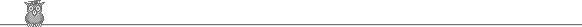### 25th Putnam 1964Problem A2

α is a real number. Find all continuous real-valued functions f :[0, 1] → (0, ∞) such that ∫01 f(x) dx = 1, ∫01 x f(x) dx = α, ∫01 x2 f(x) dx = α2.

Solution

We have ∫01 (α - x)2f(x) dx = α2 - 2α2 + α2 = 0. But the integrand is positive, except possibly for one point of the range, so the integral must also be positive. Contradiction. So there are no functions with this property.